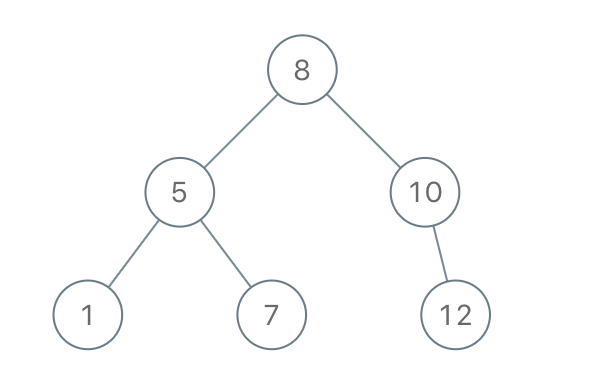# 1008. Construct Binary Search Tree from Preorder Traversal

Medium
Given an array of integers preorder, which represents the preorder traversal of a BST (i.e., binary search tree), construct the tree and return its root.
It is guaranteed that there is always possible to find a binary search tree with the given requirements for the given test cases.
A binary search tree is a binary tree where for every node, any descendant of `Node.left` has a value strictly less than `Node.val`, and any descendant of `Node.right` has a value strictly greater than `Node.val`.
A preorder traversal of a binary tree displays the value of the node first, then traverses `Node.left`, then traverses `Node.right`.
Example 1:Input: preorder = [8,5,1,7,10,12]
Output: [8,5,10,1,7,null,12]
Example 2:
Input: preorder = [1,3]
Output: [1,null,3]
Constraints:
• `1 <= preorder.length <= 100`
• `1 <= preorder[i] <= 1000`
• All the values of `preorder` are unique.

### 解題

Runtime: 0 ms, faster than 100%
Memory Usage: 2.3 MB, less than 100%
/**
* Definition for a binary tree node.
* type TreeNode struct {
* Val int
* Left *TreeNode
* Right *TreeNode
* }
*/
func bstFromPreorder(preorder []int) *TreeNode {
// preorder -> 中 左 右
if len(preorder) == 0 {
return nil
}
i := 1
for i=1; i<len(preorder); i++ {
if preorder[i] > preorder {
break
}
}
return &TreeNode{ Val: preorder, Left: bstFromPreorder(preorder[1:i]), Right: bstFromPreorder(preorder[i:]) }
}